### 吉利汽车 吉利EC8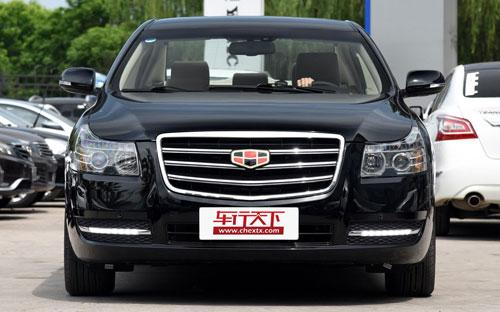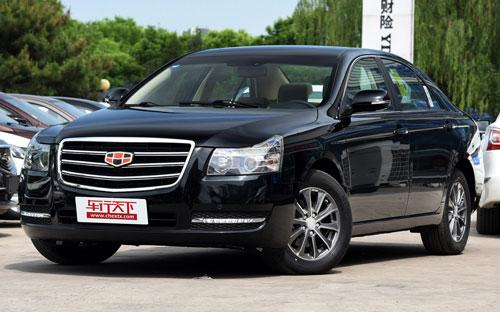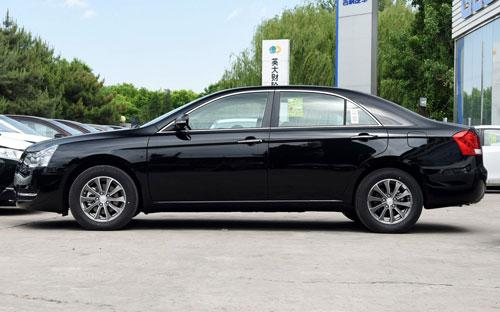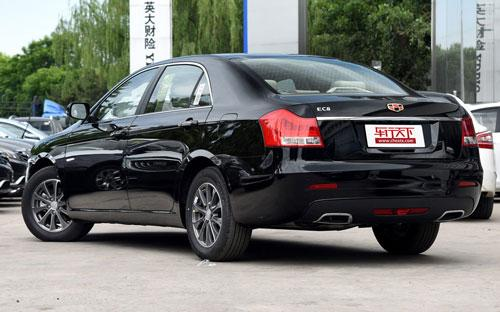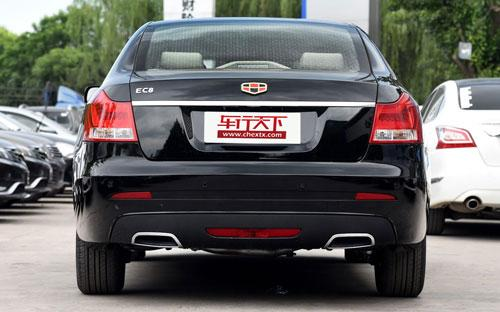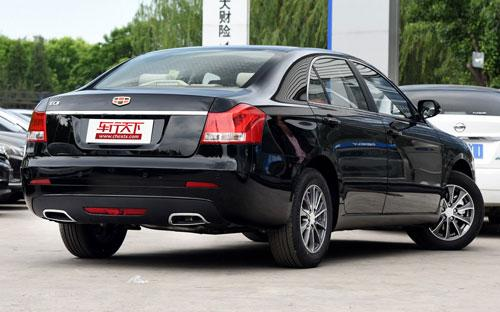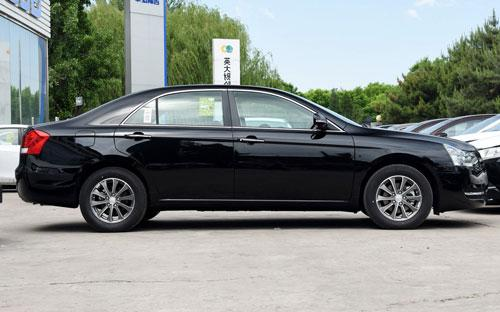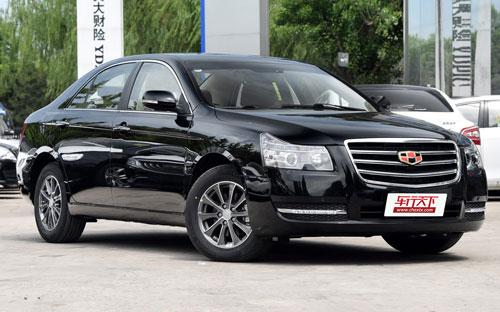4 种颜色可选2015款最低售价：8.98 万元起

4905(mm)1830(mm)1495(mm)##### 配置亮点：
• 胎压监测装置

• ISOFIX儿童座椅接口

• 车身稳定控制(ESC/ESP/DSC等)

• 电动天窗

• 定速巡航

• 后倒车雷达

• 真皮座椅

• GPS导航系统

• 氙气大灯

• 后视镜加热

• 提交
2015款 2.0L 自动精英型 (95张)

吉利汽车 吉利EC8 绕车实拍• 吉利汽车 吉利EC8 在售车型

排量 车型 厂商指导价 本地最低报价 购车工具
2.0L
8.98万
8.98万

9.89万
9.89万

2.0L 自动精英型 6挡手自一体
10.89万
10.89万

2.0L 自动尊贵型 6挡手自一体
11.89万
11.89万

2.4L
8.98万
8.98万

9.89万
9.89万

2.0L 自动精英型 6挡手自一体
10.89万
10.89万

2.0L 自动尊贵型 6挡手自一体
11.89万
11.89万

吉利汽车 吉利EC8 经销商

查看更多 >>

### 吉利汽车 吉利EC8 动力加速

吉利EC8 0-100公里加速时间分布在 0.0-秒 属于 超跑级

动力级别 加速时间 车型

吉利汽车 吉利EC8 视频

吉利汽车 吉利EC8 新闻资讯

# 2015款吉利EC8正式上市 售8.89-11.89万

国产新车 超过8806次关注

1月20日，吉利汽车宣布推出2015款吉利EC8，四款车型售价区间为8.89-11.89万元。相比老款，2015款吉利EC8取消了2.4L车型，而2.0L四款车型价格相比老款也有所下调。

# 自主中型车篇 吉利帝豪EC8车型推荐

车系文章 超过2415次关注

帝豪EC8作为吉利旗下首款中型车，从外形方面设计的还是相当稳重大气，相比其它自主品牌中型车的外形商务气息也更浓，而且看起来也要更大一些。这点上从其4905×1830...

# 北京车展静态评测帝豪EC8 配置很丰富

车系文章 超过3467次关注

第十一届北京国际车展正式拉开帷幕，众多新车的亮相让全世界的目光汇聚于此，特别是自主品牌推出的众多新车让人眼花缭乱，吉利就是其中之一。而吉利旗下帝豪品牌全...

# 测试新款帝豪EC8 用料讲究/做工细腻

试驾评测 超过4155次关注

如今国内市场上的中型车有这么几个特点：尺寸越做越大，6AT开始普及，优惠幅度也越来越大。刚上市的新款吉利帝豪EC8就符合这些特点，它有超过4.9米长的车身，超过2....

# 新款吉利EC8实车谍照曝光 有望年内推出

车系文章 超过2259次关注

近日，国内相关媒体曝光了一组新款吉利EC8的实车谍照。据此前信息，这款新车有望在今年年内推出。

# 吉利帝豪新款EC8谍照曝光 尾部设计改变

车系文章 超过2226次关注

日前，我们得到了一组吉利帝豪改款EC8的谍照，这款车有望于年内发布。

# 吉利帝豪新款EC8上市 售9.98万-20.98万

车系文章 超过1936次关注

吉利汽车旗下新款EC8正式上市，此次上市的新款EC8共有11款车型，售价区间为9.98万-20.98万元。

猜你喜欢

﻿
• 快速找车
• 选择品牌
• 选择品牌
• A  奥迪
• A  阿斯顿·马丁
• A  阿尔法·罗密欧
• B  宝沃
• B  布加迪
• B  巴博斯
• B  保时捷
• B  宾利
• B  奔驰
• B  宝马
• B  本田
• B  别克
• B  标致
• B  比亚迪
• B  宝骏
• B  北汽制造
• B  北汽新能源
• B  北汽幻速
• B  北汽威旺
• B  北京汽车
• B  奔腾
• B  北汽绅宝
• C  长安
• C  长安商用
• C  长城
• C  昌河
• D  大众
• D  道奇
• D  DS
• D  东南
• D  东风风神
• D  东风风行
• D  东风小康
• D  东风风度
• D  东风
• F  福特
• F  丰田
• F  菲亚特
• F  法拉利
• F  福田
• F  福迪
• F  福汽启腾
• G  观致
• G  广汽传祺
• G  广汽吉奥
• G  GMC
• H  红旗
• H  汉腾汽车
• H  哈弗
• H  哈飞
• H  海格
• H  海马
• H  华颂
• H  黄海
• H  华泰
• H  恒天
• J  吉利汽车
• J  捷豹
• J  Jeep
• J  江淮
• J  江铃
• J  金杯
• J  九龙
• J  金旅
• K  凯翼
• K  凯迪拉克
• K  克莱斯勒
• K  科尼塞克
• K  卡威
• K  开瑞
• L  路虎
• L  林肯
• L  劳斯莱斯
• L  兰博基尼
• L  雷克萨斯
• L  铃木
• L  雷诺
• L  理念
• L  力帆
• L  莲花汽车
• L  猎豹
• L  路特斯
• L  陆风
• M  马自达
• M  MG
• M  MINI
• M  玛莎拉蒂
• M  摩根
• M  迈凯轮
• N  纳智捷
• O  欧宝
• O  讴歌
• O  欧朗
• Q  奇瑞
• Q  起亚
• Q  启辰
• R  日产
• R  荣威
• R  瑞麒
• S  三菱
• S  斯威汽车
• S  萨博
• S  smart
• S  斯柯达
• S  斯巴鲁
• S  思铭
• S  双龙
• S  上汽大通
• S  双环
• T  特斯拉
• T  腾势
• W  沃尔沃
• W  五菱汽车
• W  五十铃
• W  威兹曼
• W  威麟
• X  现代
• X  雪佛兰
• X  雪铁龙
• X  西雅特
• Y  一汽
• Y  英菲尼迪
• Y  英致
• Y  依维柯
• Y  野马汽车
• Y  永源
• Z  众泰
• Z  中华
• Z  中兴
• Z  知豆
• 选择车系
• 选择车系
• 车型对比
• 选择品牌
• 选择品牌
• A  奥迪
• A  阿斯顿·马丁
• A  阿尔法·罗密欧
• B  宝沃
• B  布加迪
• B  巴博斯
• B  保时捷
• B  宾利
• B  奔驰
• B  宝马
• B  本田
• B  别克
• B  标致
• B  比亚迪
• B  宝骏
• B  北汽制造
• B  北汽新能源
• B  北汽幻速
• B  北汽威旺
• B  北京汽车
• B  奔腾
• B  北汽绅宝
• C  长安
• C  长安商用
• C  长城
• C  昌河
• D  大众
• D  道奇
• D  DS
• D  东南
• D  东风风神
• D  东风风行
• D  东风小康
• D  东风风度
• D  东风
• F  福特
• F  丰田
• F  菲亚特
• F  法拉利
• F  福田
• F  福迪
• F  福汽启腾
• G  观致
• G  广汽传祺
• G  广汽吉奥
• G  GMC
• H  红旗
• H  汉腾汽车
• H  哈弗
• H  哈飞
• H  海格
• H  海马
• H  华颂
• H  黄海
• H  华泰
• H  恒天
• J  吉利汽车
• J  捷豹
• J  Jeep
• J  江淮
• J  江铃
• J  金杯
• J  九龙
• J  金旅
• K  凯翼
• K  凯迪拉克
• K  克莱斯勒
• K  科尼塞克
• K  卡威
• K  开瑞
• L  路虎
• L  林肯
• L  劳斯莱斯
• L  兰博基尼
• L  雷克萨斯
• L  铃木
• L  雷诺
• L  理念
• L  力帆
• L  莲花汽车
• L  猎豹
• L  路特斯
• L  陆风
• M  马自达
• M  MG
• M  MINI
• M  玛莎拉蒂
• M  摩根
• M  迈凯轮
• N  纳智捷
• O  欧宝
• O  讴歌
• O  欧朗
• Q  奇瑞
• Q  起亚
• Q  启辰
• R  日产
• R  荣威
• R  瑞麒
• S  三菱
• S  斯威汽车
• S  萨博
• S  smart
• S  斯柯达
• S  斯巴鲁
• S  思铭
• S  双龙
• S  上汽大通
• S  双环
• T  特斯拉
• T  腾势
• W  沃尔沃
• W  五菱汽车
• W  五十铃
• W  威兹曼
• W  威麟
• X  现代
• X  雪佛兰
• X  雪铁龙
• X  西雅特
• Y  一汽
• Y  英菲尼迪
• Y  英致
• Y  依维柯
• Y  野马汽车
• Y  永源
• Z  众泰
• Z  中华
• Z  中兴
• Z  知豆
• 选择车系
• 选择车系
• 选择车型
• 选择车型
• 意见反馈